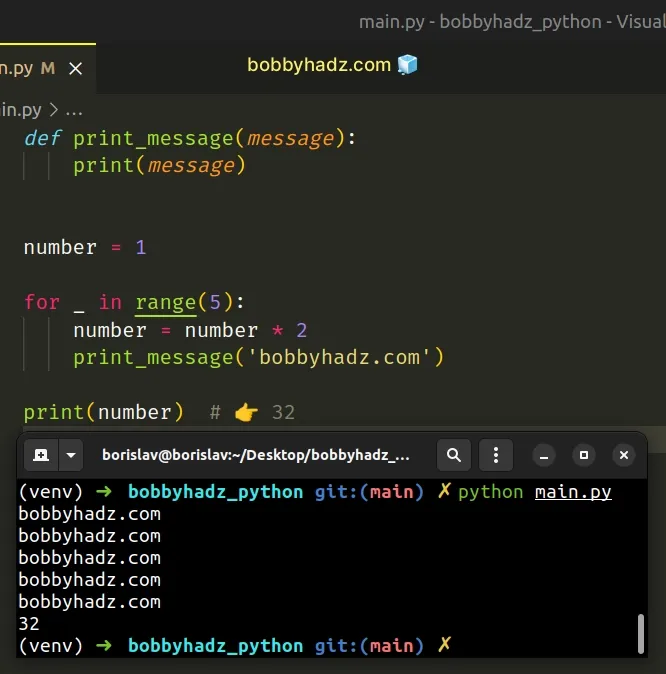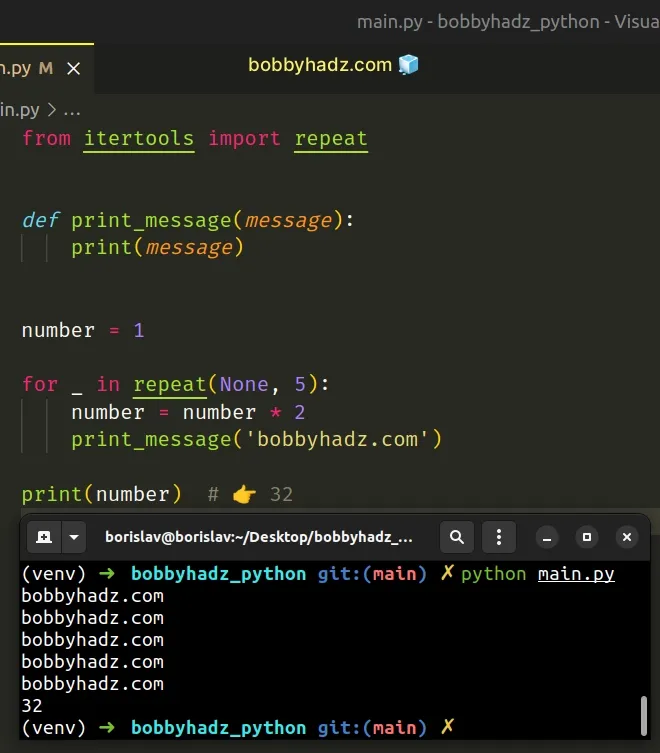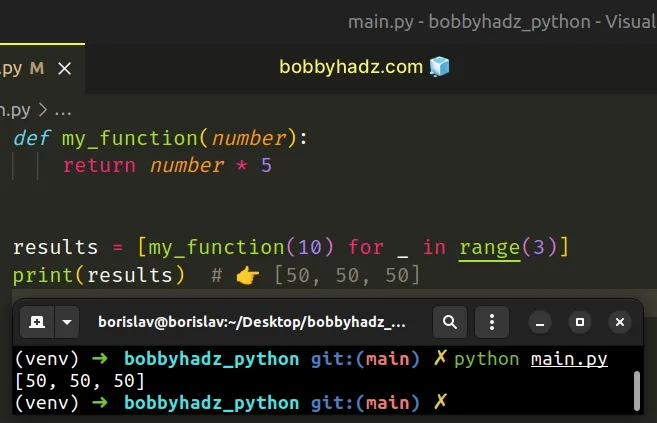# How to call a Function N times in PythonLast updated: Feb 22, 2023
4 min## #Call a function N times in Python

To call a function N times:

1. Use the `range()` class to create a `range` object of length N.
2. Use a `for` loop to iterate over the `range` object.
3. Call the function on each iteration.
main.py
```Copied!```def print_message(message):
print(message)

number = 1

for _ in range(5):
number = number * 2

print(number)  # 👉️ 32
``````We used a for loop to iterate over a `range` object N times.

The range class is commonly used for looping a specific number of times in `for` loops and takes the following arguments:

NameDescription
`start`An integer representing the start of the range (defaults to `0`)
`stop`Go up to, but not including the provided integer
`step`Range will consist of every N numbers from `start` to `stop` (defaults to `1`)

If you only pass a single argument to the `range()` constructor, it is considered to be the value for the `stop` parameter.

main.py
```Copied!```for n in range(5):
print(n) # 👉️ 0 1 2 3 4

result = list(range(5))

# 👇️ [0, 1, 2, 3, 4]
print(result)
``````
The example shows that if the `start` argument is omitted, it defaults to `0` and if the `step` argument is omitted, it defaults to `1`.

If values for the `start` and `stop` parameters are provided, the `start` value is inclusive, whereas the `stop` value is exclusive.

main.py
```Copied!```result = list(range(1, 5))

# 👇️ [1, 2, 3, 4]
print(result)
``````

On each iteration of the `for` loop, we call a function and reassign a variable.

main.py
```Copied!```def print_message(message):
print(message)

number = 1

for _ in range(5):
number = number * 2

print(number)  # 👉️ 32
``````

You can use this approach to perform any other action N times.

We used an underscore `_` for the variable's name. There is a convention to use an underscore `_` for placeholder variables we don't intend to use.

Alternatively, you can use the `itertools.repeat()` class.

## #Call a function N times using itertools.repeat()

This is a three-step process:

1. Use the `itertools.repeat()` class to create an iterator of length N.
2. Use a `for` loop to iterate over the iterator.
3. Call the function on each iteration.
main.py
```Copied!```from itertools import repeat

def print_message(message):
print(message)

number = 1

for _ in repeat(None, 5):
number = number * 2

print(number)  # 👉️ 32
``````The itertools.repeat class takes an object and the number of times the object should be repeated as arguments.

main.py
```Copied!```from itertools import repeat

# 👇️ ['x', 'x', 'x', 'x', 'x']
print(list(repeat('x', 5)))
``````

The method returns an iterator containing the object repeated N times.

The `itertools.repeat()` class is a little faster than the `range()` class in this scenario because it doesn't have to create an `int` object on each iteration.

However, the difference in performance is negligible (~15%) for most applications.

## #Call a function N times using a list comprehension

This is a two-step process:

1. Use a list comprehension to iterate over a `range` object with length N.
2. On each iteration, call the function and return the result.
main.py
```Copied!```def my_function(number):
return number * 5

results = [my_function(10) for _ in range(3)]
print(results)  # 👉️ [50, 50, 50]
``````We used a list comprehension to iterate over the `range` object.

List comprehensions are used to perform some operation for every element or select a subset of elements that meet a condition.

On each iteration, we call the function and return the result.

## #Call a function N times and store the results in a list using a `for` loop

You can also use a `for` loop to call a function N times and store the results in a list.

main.py
```Copied!```def increment(num):
return num + 100

results = []

for i in range(1, 6):
results.append(increment(i))

print(results)  # 👉️ [101, 102, 103, 104, 105]
``````

On each iteration of the `for` loop, we call the function and append the result to the list.

After the last iteration, the list contains the output of all of the function's invocations.

## #Call a function N times using map()

You can also use the `map()` function to call a function N times.

main.py
```Copied!```def increment(num):
return num + 100

list_of_arguments = [1, 2, 3, 4, 5]

results = list(map(increment, list_of_arguments))

print(results)  # 👉️ [101, 102, 103, 104, 105]
``````

The map() function takes a function and an iterable as arguments and calls the function with each item of the iterable.

We passed the `increment` function to `map`, so it gets called with each item from the list.

The last step is to use the `list()` class to convert the `map` object to a list.

## #Call a function N times using a `while` loop

You can also use a `while` loop to call a function N times.

main.py
```Copied!```def print_message(message):
print(message)

n = 3

while n > 0:

n -= 1

``````

We initialized the `n` variable to `3` and used a `while` loop to iterate for as long as the variable is greater than `0`.

On each iteration, we call the `print_message` function and decrement the `n` variable.

Once the `n` variable is set to `0` or less than `0`, the condition is no longer met and the `while` loop exits.